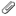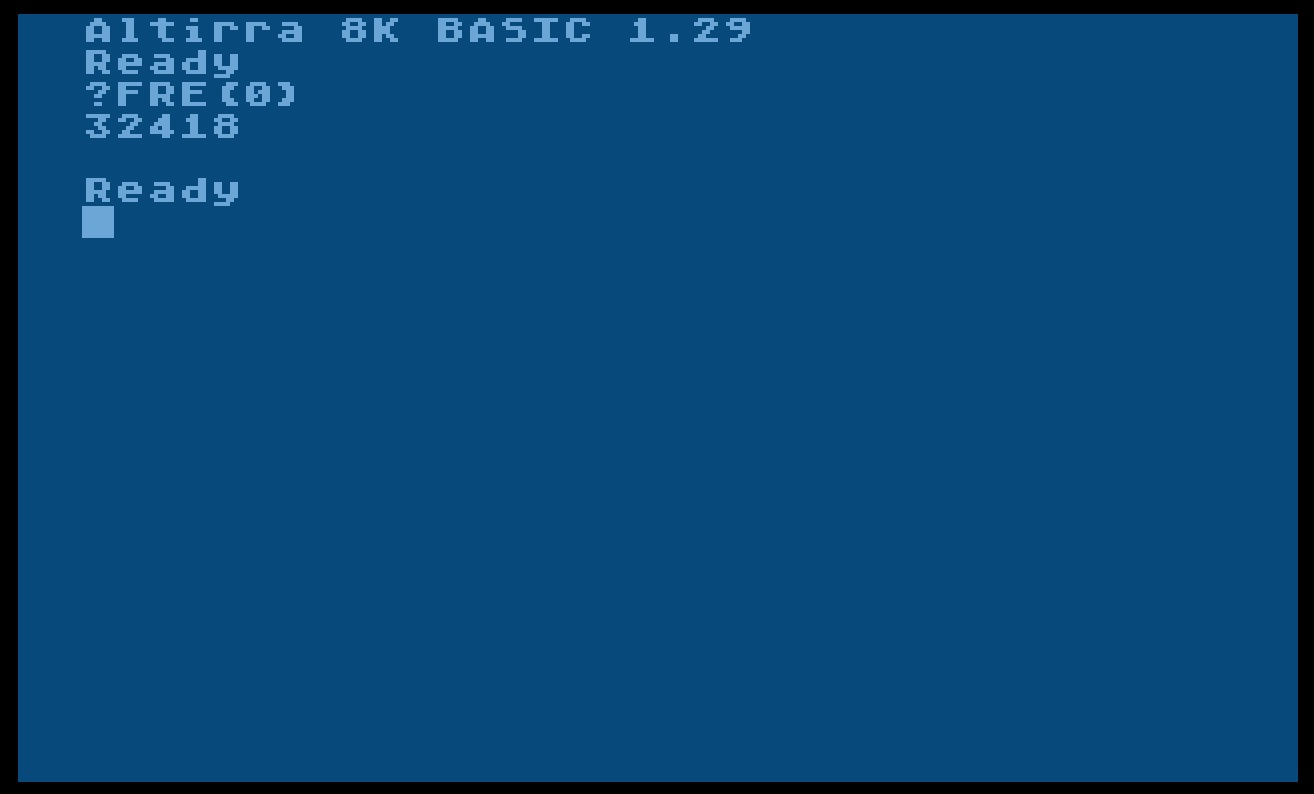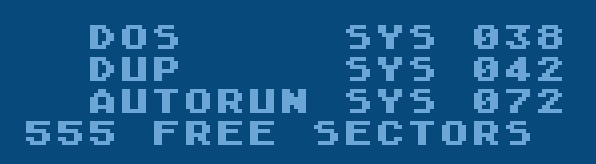This is version . It is not the current version, and thus it cannot be edited.
[Back to current version]   [Restore this version]

## Altirra BASIC#

### CAR-Image:#

Altirra BASIC 1.29: atbasic.car; size: 12 KB

### BIN-Image:#

Altirra BASIC 1.29: atbasic.bin; size: 8 KB

### ATR-Image:#

Altirra BASIC 1.29: Altirra Basic 1.29 with DOS II 2.5.atr; ATR-Image with DOS 2.5 and Altirra BASIC 1.29 as AUTORUN.SYS file

### Images:#Altirra BASIC 1.29 after starting from the ATR-imageSectors needed for Altirra BASIC 1.29 on the SD-ATR-image above

### Reference:#

The original, actual version and the source code of Altirra Basic can be found can be found here:

Altirra at virtualdub.orgThe source code itself is published within the Altirra source code package (src/ATBasic/*). For the license please see at the top of the page.

### Manual:#

Avery has given us the following infos:
a)
Added CP, HSTICK() and VSTICK() to ATBasic, then found a bunch of bugs to fix, like bitwise operators not listing properly and a regression in nested precedence handling. Extensions supported: \$hhhh, %, !, &, HEX\$(), DPEEK(), HSTICK(), VSTICK(), DPOKE, BPUT, BGET, CP, ERASE, PROTECT, UNPROTECT, DIR, RENAME, MOVE.
b)
From the tokens.inc-file we find:
Altirra BASIC - Token definitions
Copying and distribution of this file, with or without modification, are permitted in any medium without royalty provided the copyright notice and this notice are preserved. This file is offered as-is, without any warranty.

TOK_EOS = \$14 ;end of statement
TOK_EOL = \$16 ;end of line

TOK_REM = \$00
TOK_DATA = \$01
TOK_INPUT = \$02
TOK_COLOR = \$03
TOK_LIST = \$04
TOK_ENTER = \$05
TOK_LET = \$06
TOK_IF = \$07
TOK_FOR = \$08
TOK_NEXT = \$09
TOK_GOTO = \$0A
TOK_GOTO2 = \$0B
TOK_GOSUB = \$0C
TOK_TRAP = \$0D
TOK_BYE = \$0E
TOK_CONT = \$0F
TOK_COM = \$10
TOK_CLOSE = \$11
TOK_CLR = \$12
TOK_DEG = \$13
TOK_DIM = \$14
TOK_END = \$15
TOK_NEW = \$16
TOK_OPEN = \$17
TOK_SAVE = \$19
TOK_STATUS = \$1A
TOK_NOTE = \$1B
TOK_POINT = \$1C
TOK_XIO = \$1D
TOK_ON = \$1E
TOK_POKE = \$1F
TOK_PRINT = \$20
TOK_RESTORE = \$23
TOK_RETURN = \$24
TOK_RUN = \$25
TOK_STOP = \$26
TOK_POP = \$27
TOK_QMARK = \$28
TOK_GET = \$29
TOK_PUT = \$2A
TOK_GRAPHICS= \$2B
TOK_PLOT = \$2C
TOK_POSITION= \$2D
TOK_DOS = \$2E
TOK_DRAWTO = \$2F
TOK_SETCOLOR= \$30
TOK_LOCATE = \$31
TOK_SOUND = \$32
TOK_LPRINT = \$33
TOK_CSAVE = \$34
TOK_ILET = \$36
TOK_SXERROR = \$37

TOK_EXP_CNUM = \$0E ;constant float number
TOK_EXP_CSTR = \$0F ;constant string
TOK_EXP_COMMA = \$12 ;comma
TOK_EXP_SEMI = \$15 ;semicolon
TOK_EXP_GOTO = \$17
TOK_EXP_GOSUB = \$18
TOK_EXP_TO = \$19
TOK_EXP_STEP = \$1A
TOK_EXP_THEN = \$1B
TOK_EXP_HASH = \$1C
TOK_EXP_LE = \$1D
TOK_EXP_NE = \$1E
TOK_EXP_GE = \$1F
TOK_EXP_LT = \$20
TOK_EXP_GT = \$21
TOK_EXP_EQUAL = \$22
TOK_EXP_POWER = \$23
TOK_EXP_MULTIPLY = \$24
TOK_EXP_SUBTRACT = \$26
TOK_EXP_DIVIDE = \$27
TOK_EXP_NOT = \$28
TOK_EXP_OR = \$29
TOK_EXP_AND = \$2A
TOK_EXP_OPENPAREN = \$2B
TOK_EXP_CLOSEPAREN = \$2C
TOK_EXP_ASSIGNNUM = \$2D
TOK_EXP_ASSIGNSTR = \$2E
TOK_EXP_STR_LE = \$2F
TOK_EXP_STR_NE = \$30
TOK_EXP_STR_GE = \$31
TOK_EXP_STR_LT = \$32
TOK_EXP_STR_GT = \$33
TOK_EXP_STR_EQ = \$34
TOK_EXP_UNPLUS = \$35 ;unary plus
TOK_EXP_UNMINUS = \$36 ;unary minus
TOK_EXP_OPEN_STR = \$37 ;open parens, string array
TOK_EXP_OPEN_ARY = \$38 ;open parens, numerical array
TOK_EXP_OPEN_DIMARY = \$39 ;open parens, dim numerical array
TOK_EXP_OPEN_FUN = \$3A ;open parens, function
TOK_EXP_OPEN_DIMSTR = \$3B ;open parens, dim string array
TOK_EXP_ARRAY_COMMA = \$3C ;array subscript comma

Only authorized users are allowed to upload new attachments.

### List of attachments

Kind Attachment Name Size Version Date Modified Author Change note
atr
atr
Altirra Basic 1.29 with DOS II... 92.2 kB 1 18-Nov-2014 21:12 Roland B. Wassenberg ATR-Image with AUTORUN.SYS
jpg
Altirra1.jpg 53.5 kB 1 18-Nov-2014 21:13 Roland B. Wassenberg figure 1
jpg
Altirra2.jpg 27.8 kB 1 18-Nov-2014 21:13 Roland B. Wassenberg figure 2
bin
atbasic.bin 8.2 kB 1 18-Nov-2014 21:12 Roland B. Wassenberg BIN-Image
car
atbasic.car 8.2 kB 1 18-Nov-2014 21:11 Roland B. Wassenberg Cartridge
« This particular version was published on 18-Nov-2014 23:33 by Roland B. Wassenberg.
G’day (anonymous guest) My Prefs
Articles
Hardware
Software
Applications
Memory Map Topic list
MicroUSB
AllNews
FAQ
Index
Languages
Code

What's New?

Voice and Data Cassettes
Atari Program Exchange (APX)
Atari Learning System Software
PLATO (Programmed Logic for Automated Teaching Operation)
Official country specific Atari software
• Belgium-Netherlands-Luxembourg• France• Germany• Italy• United KingdomThanks
Rarity 10
Statistics
All content in the Wiki is licensed under Creative Commons Share Alike License, unless otherwise noted.